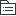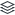# 高中数学

|
71

## 相关模板推荐

•## 数学 —— 作品大纲

•算术
• 整数
• 实数
• 比与比例
• 数轴与绝对值
•代数
• 整式
• 分式
• 函数
• 代数方程
• 不等式
• 数列
• 基础概念
• 数列定义
• 按一定的次序排列的有规律的一列数
• 通项
• a(n)=f(n)
• 前n项和
• s(n)
• a(n)与s(n)的关系
• a(n)=
• s1 n=1
• sn=s(n-1) n>=2
• 等差等比数列
• 等差数列
• 核心元素
• a1、d
• 定义
• a(n+1)-an=d
• 常数列为特殊等差数列
• 通项
• an=a1+(n-1)d
• 关于n的一次函数
• 三项
• 数列a、b、c成等差数列
• b=(a+c)/2
• 角标
• 如果m+n=s+t
• am+an=as+at
• 求和
• sn=(n(a1+an))/2
• sn=na1+(n(n-1))/2*d
• 性质
• sn，s(2n)-sn，s(3n)-s(2n),#####仍成等差数列
• 等比数列
• 核心元素
• a1、q
• 定义
• a(n+1)/an=q
• 非0常数列为特殊等比数列
• 通项
• an=a1*q^(n-1)
• 关于n的指数函数
• 三项
• 数列a、b、c成等比数列
• b^2=ac
• 角标
• 如果m+n=s+t
• am*an=as*at
• 求和
• sn
• (a1(1-q^n))/(1-q) q不为1
• na1 q=1
• 若|q|<1，则该数列的所有项和a1/(1-q)
• 性质
• sn，s(2n)-sn，s(3n)-s(2n),#####仍成等比数列
• 等差等比数列通项问题
• 设a1，d或a1，q
• 等差数列
• an=a1+(n-1)d=dn+(a1-d)
• an=am+(n-m)d
• 等比数列
• an=a1q^(n-1)
• an=amq^(n-m)
• 等差、等比脚注性质与中项公式
• 角标性质
• 角标和相等且对应项数也相等
• 三项问题
• 经验
• 数列a，b，c成等差且等比
• a=b=c
• 注意
• 等比数列项的正负性
• 总结
• 等差数列
• 已知和
• 三项
• a-d，a，a+d
• 四项
• a-3d，a-d，a+d，a+3d
• 等比数列
• 已和积
• 三项
• a/q，a，aq
• 四项
• a/(q^3)，a/q，aq，aq^3
• 等差数列求和问题
• 公式1
• sn=(n(a1+an))/2
• 平均数*项数
• 角标性质*对数
• 公式2
• sn=na1+(n(n-1))/2*d
• 注意
• 等差{an}，{bn}的前n项和分别为sn与tn，则ak/bk=s(2k-1)/t(2k-1)
• 等差数列sn图像、最值、变号问题
• 图像sn
• sn=na1+(n(n-1))/2*d=
• d/2*n^2+(a1-d/2)n
• 关于n的一个二次函数，且常数项为0
• d>0
• 有最小值
• d<0
• 有最大值
• sn最值问题
• 方法1
• 从通项an角度
• a1<0，d>0
• sn有最小值
• an<=0，a(n+1)>0
• 求出n
• 方法2
• 从sn二次函数角度
• sn=na1+(n(n-1))/2*d=d/2*n^2+(a1-d/2)n
• 对称轴n对=-b/(2a)=-(a1-d/2)/(2*d/2)=1/2-a1/d
• 特别注意
• 把sn图形理解成二次函数分析
• 等差sn变号问题
• sn=((a1+an)n)+角标性质分析
• 等比数列求和
• sn
• (a1(1-q^n))/(1-q)=（a1-anq）/(1-q) q不为1
• na1 q=1
• 注意
• sn
• a1/(1-q)-(a1/(1-q))*q^n
• =k-kq^n
• 非等差等比数列
• 思路
• 化归到等差等比数列
• 穷举找规律
• 总结
• 转化为等差等比
• 穷举找规律
• 数列应用题
• 经验
• 区分等差等比数列
• 区分求an
• 注意项数
• 总结
• 等差等比化归思想
• 穷举找规律思路
• 特值法
• 常数数列
•几何
• 平面几何
• 三角形面积计算
• 1/2*底*高
• 边之比===面积之比
• 三角函数
• 利用全等或相似
• 三角形的四心
• 内心
• 角平分线
• 三角形内切圆的圆心
• 外心
• 三边垂直平分线
• 三角形外接圆的圆心
• 重心
• 三边中线
• 垂心
• 三边高
• 特殊三角形
• 直角三角形、等腰三角形、等腰直角三角形、等边三角形
• 两个三角形全等或相似
• 全等
• 旋转、折叠找 全等
• 相似
• 平行直接找相似
• 直角三角形射影定理
• CB^2=BD*AB
• 四边形
• 添半径
• 组合图形
• 直角三角形的内切圆与外接圆
• 内切圆
• r=(a+b-c)/2
• 外接圆
• R=c/2
• 圆套圆与方套方
• 圆套圆
• 圆方圆
• 面积之比=2*3.14：4：3.14
• 方套方
• 方圆方
• 面积之比=4：3.14：2
• 平面几何专题
• 不规则圆形阴影面积问题
• 用割补发把不规则图形转化为规则图形
• 注意
• 熟悉与圆有关的一些图形
• 图形的重叠、对称、平移
• 总结
• 平面几何应试技巧
• 量，有图有真相
• 量答案
• 加条件
• 三角形加高
• 四边形加高或平行线
• 圆加半径
• 中点中外线
• 转图形再看
• 特殊化
• 立体几何
• 长方体、正方体
• 体积
• V=abc
• 表面积
• S=2(ab+ac+bc)
• 体对角线
• L=(a^2+b^2+c^2)的平方根
• 公式经验结论
• FIRST
• (ab)(ac)(bc)=(abc)^2
• 三个不同面的面积之积=（体积）^2
• (a+b+c)^2=((a^2+b^2+c^2)d的平方根)^2+2(ab+ac+bc)
• (1/4棱长之和)=（L）^2+表面积
• ((a^2+b^2)的平方根)^2+((a^2+c^2)的平方根)^2+((c^2+c^2)的平方根)^2
• =2((a^2+b^2+c^2)的平方根)^2
• 三个面对角线的平方和=2（L）^2
• SECOND
• 涂色
• 一个表面涂红色正方体切成N^3
• 三面涂色的有8块
• 8个顶点
• 二面涂色的有12（N—2）块
• 一面涂色的有6（N-2）^2块
• 无涂色的（N-2^3）
• 最短路
• a<b<c
• l最短=（（a+b）^2+c^2）的平方根
• 物体位于体对角线两侧
• 展开图
• 拼接
• 切多2面
• 接少2面
• 圆柱体
• 基本公式
• V=3.14*r^2*h
• S侧=2*3.14*r*h
• S全=2*3.14*r*h+2*3.14*r^2
• 球体
• V=4/3*3.14*r^3
• V表=4*3.14*r^2
• 立体几何专题
• 内切圆与外接圆
• 长方体
• 没有内切圆
• L=2R外
• 正方体
• 2r内=a
• R外=3的平方根/2*a
• 等边圆柱
• r=r内
• L=2R外
• 关键
• 直径=边长
• 直径=体对角线
• 平面解析几何
• 用代数方式处理平面几何问题
• 特点
• 公式多，计算烦，两解多
• 一般方法
• 待定系数法
• 实用技巧
• 反向验证
• 画草图定性判断
• 巧解特殊三角形
• 特殊对称
• 基本知识
• 四大公式
• 中点
• （(x1+x2)/2,(y1+y2)/2）
• 距离
• ((x2-x1)^2+(y2-y1)^2)的平方根
• 点到直线距离
• d=|Ax0+By0+C|/((A^2+B^2)的平方根)
• 斜率
• 定义式k=夹角的正切
• k=(y2-y1)/(x2-x1)
• 注意
• x=a 夹角为90度，k不存在
• 大小比较
• |k|越大，直线越贴近y轴
• 取值范围
• 直线方程
• 五种表达式
• y=kx+b
• y-y1=k(x-x1)
• (y-y1)/(y2-y1)=(x-x1)/(x2-x1)
• x/a+y/b=1
• Ax+By+C=0
• 求直线方程
• 设k，可能会漏解x=a
• 在两坐标轴上截距相等
• x/a+y/a=1
• y=kx
• x/a+y/b=1与坐标轴所围三角形的面积
• S=1/2|ab|
• 一般式
• x^2+y^2+Dx+Ey+F=0
• 表示圆<====>D^2+E^2-4F>0
• x^2与y^2的系数相等
• 圆心为（-D/2,-E/2）
• 半径为(（D^2+E^2-4F）/4)的平方根
• 标准式
• (x-x0)^2+(y-y0)^2=r^2
• 圆心（x0，y0）
• 半径为r
• 位置关系
• 点与直线的关系
• 两直线的关系
• 设两直线l1:y=k1x+b1，l2:y=k2x+b2
• 相交k1不等于k2
• 平行k1=k2且b1不等于b2
• 重合k1=k2且b1=b2
• 垂直k1*k2=-1
• A1A2*B1B2=0
• 两直线间的距离d=|C1-C2|/（(A^2+B^2)的平方根）
• 直线系（过定点）方程
• (A1x+B1y+C1)+(A2x+B2y+C2)z=0
• 必过定点
• A1x+B1y+C1=0
• A2x+B2y+C2=0
• 直线与圆的关系
• 直线l:Ax+By+C=0，圆（x-a）^2+（y-b）^2=r^2
• 那么圆心M（a,b）到直线l的距离
• d=|Aa+Bb+C|/((A^2+B^2)的平方根)
• 直线与圆相离
• d>r
• 最短距离=d-r
• 最远距离=d+r
• 直线与圆相切
• d=r
• 求圆的切线x^2+y^2=a^2
• 切点在圆上
• xx0+yy0=r^2
• 点在圆外
• 必有两条（会漏掉x=a的直线）
• 直线与圆相交
• d<r
• 弦长AB=2（（r^2-d^2）平方根）
• 弦长问题
• 弦长AB=2（（r^2-d^2）平方根）
• 点与圆的关系
• 点（X0,y0），圆（x-a）^2+（y-b）^2=r^2
• 在圆上
• 圆（x0-a）^2+（y0-b）^2=r^2
• 在圆外
• 圆（x0-a）^2+（y0-b）^2>r^2
• 在圆内
• 圆（x0-a）^2+（y0-b）^2<r^2
• 两圆的关系
• 圆C1:（x-a1）^2+（y-b1）^2=r1^2,圆C2:（x-a2）^2+（y-b2）^2=r2^2
• 两圆的圆心距d=|C1C2|
• 相交
• |r1-r2|<d<r1+r2
• 相交弦长方程：C1-C2
• 相切
• 外切
• d=r1+r2
• 内切
• d=|r1-r2|
• 外离
• d>r1+r2
• 圆C1内含在圆C2内
• 0<=d<r2-r1
• 解析几何与平面几何综合问题
• 用解析几何语言给出图形
• 求平面几何问题
• 巧解特殊三角形
• 对称问题
• 五种特殊对称
• 方式
• 点P（x，y）
• 曲线F（x，y）=0
• Ax+By+C=0
• 关于原点
• （-x，-y）
• F（-x，-y）=0
• A(-x)+B(-y)+C=0
• 关于x轴
• （x，-y）
• F（x，-y）=0
• Ax+B(-y)+C=0
• 关于y轴
• （-x，y）
• F（-x，y）=0
• A(-x)+By+C=0
• 关于y=x
• （y，x）
• F（y，x）=0
• Ay+Bx+C=0
• 关于y=-x
• （-y，-x）
• F（-y，-x）=0
• A(-y)+B(-x)+C=0
• 点P（x0，y0）关于直线x+y+m=0的对称点
• P'（-y0-m，-x0-m）
• 点P（x0，y0）关于直线x-y+m=0的对称点
• P'（y0-m，x0+m）
• 画图记忆
• 点关于直线对称
• L0将P1P2连线垂直平分
• k(L)*k(P1P2)=-1
• P1与P2之中点在L0上
• 两相交直线对称
• 三线共点
• 夹角相等
• 平移
• 移动一个特殊点验证
• 左加右减，上加下减
• 动点问题
• 最值问题
• 轨迹
• 中点条件：传点
• 利用平面几何知识
•数据分析
• 计数原理与古典概型
• 图文并茂，动静结合
• 五大方法
• 有限少量穷举归纳
• 用字典序，避免重漏
• 区分排列还是组合
• 排列有序，组合无序
• 准确分类合理分步
• 分类方法，分步顺序
• 正难则反除法消序
• 减法除法，去不满足
• 特殊条件优先解决
• 元素位置，位置优先
• 计数
• 情况不多
• 直接穷举
• 情况个数不多
• 排列与组合用不上
• 其他数学知识点综合的问题
• 情况多
• 排列组合
• 摸球问题
• 摸球的方式
• 一次性取（无序）
• 组合
• 每次取1个（有序）
• 不放回
• 样本减少
• 放回
• 样本不变
• 独立的
• “一次性取k个球”与“逐次无放回取k个球”
• 对应结果相同，概率相同
• 经验结论
• 抽签中奖与次序无关
• 袋中有a个中奖的球，b个不中奖的球
• 逐次无放回抽，则第k次抽到中奖球的概率
• a/(a+b)与k无关
• 前面的k-1不明确
• 特例：a=1，第k次抽到中奖球的概率为1/（a+b）
• 取样问题
• 注意：含与不含；或与且；最大与最小
• 对象均不同
• 分房问题
• 背景
• 人——每人只能选一间房子
• 房——房间内可容纳0——N人
• 经验
• n人可进N个房（n<=N）共N^n种（房间数^人数）
• n人分别进N个房（n<=N）共{N*(N-1)*(N-2)……}每房间一人
• 注意
• “恰”要选；“指定”不用选
• 房间中的人数：0，1，2，3……n
• 区分“人”与“房”
• 几何问题
• 与几何有关的计数/概率
• 平面、解析、空间——古典概率
• 几何概型
• 样本点无限个
• 等可能的
• 排队问题
• 排队
• 直排
• 特殊要求
• 位置优先
• 相邻等间隔，小团体
• 打包处理——注意包内的顺序
• 不相邻问题——抽空处理
• 注意两头的空
• 定序要求
• 除法消序
• 特殊优先
• 两排问题——直排处理
• 三元素以上不相邻只能用抽空
• 环排
• n个人环排有（n-1）!种排法
• 无首无尾，方向无差别
• 分组分派问题
• 背景
• 分组元素不同的，分派的对象
• 相同
• 只分组
• 不同
• 要分派
• 注意
• 先分组再分派
• 分组：平均/非平均分
• 分派：定向/非定向
• n个组的个数一样，总数/n!
• 组数问题
• 背景
• 三位数，四位数
• 要求
• 0不能首位
• 个位：奇数/偶数，整除
• 定序
• 不对应、配对问题
• 二、三、四元素不对应分别是1，2，9种
• 涂色问题
• 乘法原理
• 某一步如果不确定===>分类
• 加法原理
• 按使用颜色个数来分类
• 相同指标不同对象分配问题——隔板处理
• 概率
• 事件间的关系+概率性质和计算
• 关系
• AB、A并B、A-B=A（-B）
• 公式
• 0<=P（A）<=1
• 对立公式
• P(-A)=1-P(A)
• P(A)=1-P(-A)
• 减法
• P(A-B)=P(A(-B))
• =P(A)-P(AB)
• 特别地
• B属于A
• ===>P(B)<=P(A)
• 0<=P(A-B)=P(A)-P(B)
• 加法
• 两事件
• P(A+B)=P(A)+P(B)-P(AB)
• 特别地
• A与B互不相容时，P(A+B)=P(A)+P(B)
• 三事件
• P(A+B+C)=P(A)+P(B)+P(C)-P(AB)-P(AC)-P(BC)+P(ABC)
• 解题思路
• 第一步事件的表示
• 利用概率公式或文氏图求概率
• 独立性的判定
• 两事件A、B
• 关系
• A、B互不相容
• A、B对立
• A、B独立
• 定义
• AB=空集
• AB=空集且A并B=U
• P(AB)=P(A)*P(B)
• 概率意义
• A、B不能同时发生
• A、B不能同时发生；A、B必有一个发生
• A事件发生对B事件发生的概率无影响
• 三事件A、B、C
• 两两对立
• 相互对立
• P(AB)=P(A)P(B)
• A、B、C相互独立
• P(AC)=P(A)P(C)
• P(BC)=P(B)P(C)
• 三三独立
• P(ABC)=P(A)P(B)P(C)
• 经验
• 若A、B、C相互独立
• 则A-B与C独立
• 则A-B与BC不一定独立
• 利用独立性计算概率
• P(AB)=P(A)P(B)
• 关键：复杂事情的分析
• n重伯努利概型
• 要点
• 每次实验只有2个结果
• 各种实验中P(A)=p，P（-A）=1-p
• n次实验是相互独立的
• 四种计算
• n次实验中A恰好发生k次的概率p
• p=(组合n、k)*p^k(1-p)^(n-k) k=0,1,2,……n
• n次实验中A至少发生1次的概率p
• p=1-(1-p)^n
• n次实验中A至多发生1次的概率p
• p=1-(1-p)^n+((组合n、1)p^1(1-p)^(n-1)
• 0次、1次
• 直到第n次实验A才发生了k次的概率p
• p={（(组合（n-1）、（k-1））}p^(k-1)（1-p）^(n-k)*p
• ={（(组合（n-1）、（k-1））}p^k（1-p）^(n-k)
• 数据分析
• 统计量——平均数与方差
• 定义与意义
• 平均数
• 算术平均数
• （x1+x2+……xn）/n
• 中心位置（对称性）
• 几何平均数
• (x1*x2*x3……xn)的n次方根
• 方差
• S^2=1/n((x1-x平)^2+(x2-x平)^2+(x3-x平)^2……(xn-x平)^2)
• 分散程度
• 标准差
• （S^2）的二次方根=S
• 注意：两组数据比较
• 平均数计算
• 扣定义
• 加权平均数
• 巧用对称性
• 方差
• 线性变换后数据的均值与方差、标准差
• 平均数y平=ax平+b
• 方差S(y)^2=a^2S^2
• 标准差（a^2S^2）的平方根=|aS|=|a|S
• 连续5个整数的方差必为2
• 多组数据的比较：异同，统计意义
• 统计 图表
• 饼图
• 可量角计算
• 频率分布直方图
• 小距形面积=各组的频率
• 各小矩形面积之和=1
•应用题
• 概述
• 一般方法
• 列方程、不等式应用题
• 应试方法
• 小学生方法
• 条件处理
• 声东击西、多个条件反向验证、条件可以特殊化、比较列表格
• 应用题中不定方程与不等式
• 未知量个数大于方程个数
• 注意
• 整数解的不定方程，用下列方法可以减少穷举的次数
• 从系数绝对值大的入手
• 利用整除性，奇偶性
• 实际问题表量有范围
• 价格问题
• 利润率=利润/进价*100%=（售价-进价）/进价*100%
• 售价=进价*（1-利润率）
• 经验
• 甲、乙以相同的售价卖出，一件赚了P%,一件亏了P%，则最终一定是亏了
• 平均问题
• 平均数=总分/总人数
• 不等式与线性规划应用题
• 不等式问题
• 线性规划应用题
• 实数解线性规划问题
• 整数解线性规划问题（穷举法）
• 直线形式
• 目标函数
• 约束条件
• 经验结论
• 最值一般在边界
• 斜率的比较
• 总结
• 线性规划解题思路
• 一般方法
• 由斜率比较大小找最优解
• 实用技巧
• 最值一般在约束条件边界点附件找到
• 集合计数
• 标数
• 用公式
• 两集合
• n(A并B)=n(A)+n(B)-n(AB)
• 三集合
• n(A并B并C)=n(A)+n(B)+n(C)-n(AB)-n(AC)-n(BC)+n(ABC)
• 函数图形应用+分段函数
• 函数图形 截距，距离
• 分段函数
• 注意：落入那一段，分段计算
• 至少至多问题
• 方法1
• >=/<=
• 不等式/最值
• 方法2
• 总量一定
• 对立面的最值
• X Y Z=总量一定
• YZ最大，则X求至少=最小
• 方法3
• 整数解
• 平均与极端思想
• 抽屉原则
• 至少有一个盒子中不少于2个球
• a只球b只盒子，至少有1个盒子不少于a/b的商加1
• 最不利原理
• 极端
• 最值应用题
• 二次函数
• 均值不等式